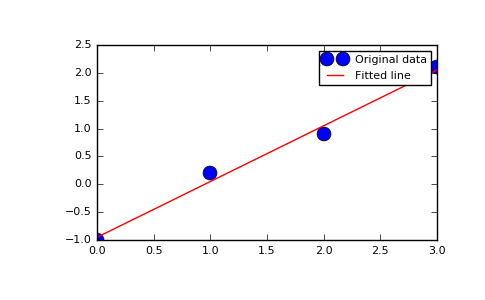# numpy.linalg.lstsq¶

`numpy.linalg.``lstsq`(a, b, rcond=-1)[source]

Return the least-squares solution to a linear matrix equation.

Solves the equation a x = b by computing a vector x that minimizes the Euclidean 2-norm || b - a x ||^2. The equation may be under-, well-, or over- determined (i.e., the number of linearly independent rows of a can be less than, equal to, or greater than its number of linearly independent columns). If a is square and of full rank, then x (but for round-off error) is the “exact” solution of the equation.

Parameters: a : (M, N) array_like “Coefficient” matrix. b : {(M,), (M, K)} array_like Ordinate or “dependent variable” values. If b is two-dimensional, the least-squares solution is calculated for each of the K columns of b. rcond : float, optional Cut-off ratio for small singular values of a. For the purposes of rank determination, singular values are treated as zero if they are smaller than rcond times the largest singular value of a. x : {(N,), (N, K)} ndarray Least-squares solution. If b is two-dimensional, the solutions are in the K columns of x. residuals : {(), (1,), (K,)} ndarray Sums of residuals; squared Euclidean 2-norm for each column in `b - a*x`. If the rank of a is < N or M <= N, this is an empty array. If b is 1-dimensional, this is a (1,) shape array. Otherwise the shape is (K,). rank : int Rank of matrix a. s : (min(M, N),) ndarray Singular values of a. LinAlgError If computation does not converge.

Notes

If b is a matrix, then all array results are returned as matrices.

Examples

Fit a line, `y = mx + c`, through some noisy data-points:

```>>> x = np.array([0, 1, 2, 3])
>>> y = np.array([-1, 0.2, 0.9, 2.1])
```

By examining the coefficients, we see that the line should have a gradient of roughly 1 and cut the y-axis at, more or less, -1.

We can rewrite the line equation as `y = Ap`, where `A = [[x 1]]` and `p = [[m], [c]]`. Now use `lstsq` to solve for p:

```>>> A = np.vstack([x, np.ones(len(x))]).T
>>> A
array([[ 0.,  1.],
[ 1.,  1.],
[ 2.,  1.],
[ 3.,  1.]])
```
```>>> m, c = np.linalg.lstsq(A, y)
>>> print(m, c)
1.0 -0.95
```

Plot the data along with the fitted line:

```>>> import matplotlib.pyplot as plt
>>> plt.plot(x, y, 'o', label='Original data', markersize=10)
>>> plt.plot(x, m*x + c, 'r', label='Fitted line')
>>> plt.legend()
>>> plt.show()
```#### Previous topic

numpy.linalg.tensorsolve

numpy.linalg.inv